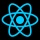Home Communities
IT Knowledge
Inspiration
Languages
EN

# Python - sort list

0 points
Created by:Admin Dirask Community
3000

In this article, we would like to show you how to sort list in Python.

Quick solution:

``````my_list = ['C', 'A', 'B']

my_list.sort()

print(my_list)  # ['A', 'B', 'C']``````

## 1. Ascending

### Example 1

In this example, we sort the list of letters in alphabetical order.

``````letters = ['C', 'A', 'B']

letters.sort()

print(letters)  # ['A', 'B', 'C']``````

Output:

``['A', 'B', 'C']``

### Example 2

In this example, we sort the list of numbers in ascending order.

``````numbers = [3, 1, 2]

numbers.sort()

print(numbers)  # [1, 2, 3]``````

Output:

``[1, 2, 3]``

## 2. Descending

### Example 1

In this example, we sort the list of letters in reverse order.

``````letters = ['A', 'C', 'B']

letters.sort(reverse=True)

print(letters)  # ['C', 'B', 'A']``````

Output:

``['C', 'B', 'A']``

### Example 2

In this example, we sort the list of numbers in descending order.

``````numbers = [3, 1, 2]

numbers.sort(reverse=True)

print(numbers)  # [3, 2, 1]``````

Output:

``[3, 2, 1]``
Join to our subscribers to be up to date with content, news and offers.